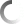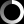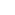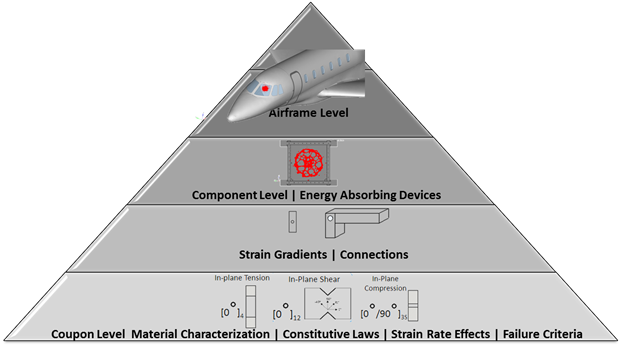## Physics Based Modeling of Dynamic Impact Events

- Non-Physics Based Modeling:

– This approach has been used by the aerospace industry since the introduction of simulation due to limitations in computing power and computational tools, complexity of the problems, poor understanding of the physics, lack of test-to-test variability data, and poor modeling methodologies.

– Simulation follows system level testing. Hence models are not predictable.

– Testing results are used to calibrate the model [non-physics based].

– Models are evaluated by the calibration-validation methods.

– The validation criteria is always unreasonable (5 to 10 %) and vague (peak, shape, subjective)  due to the lack of research and understanding of the real test-to-test variability.

- Physics Based Modeling:

– This approach used by VIMOTECH takes advantage of the advances in computational power, the latest computational tools, years of research to understand the physics, generated test-to-test variability data, and verified & validated (V&V) modeling methodologies.

– Defined modeling methodologies using the building block approach. Understanding of the physics and testing variability from the coupon to the system level. Taking a conservative modeling approach based on data derived from R&D and the Building Block Approach to define simplified models when required. The definition of the numerical model is not driven by system level test results, is driven by a predefined V&V modeling methodology.

– Simulation predicts system level test results within the scope and scatter of the physical test results.

– Objective validation criteria based on an understanding of the test-to-test variability. Defined objective validation metrics (i.e. Sprague and Gears). The correlation level between simulation and testing is driven by an understanding of the test-to-test variability.